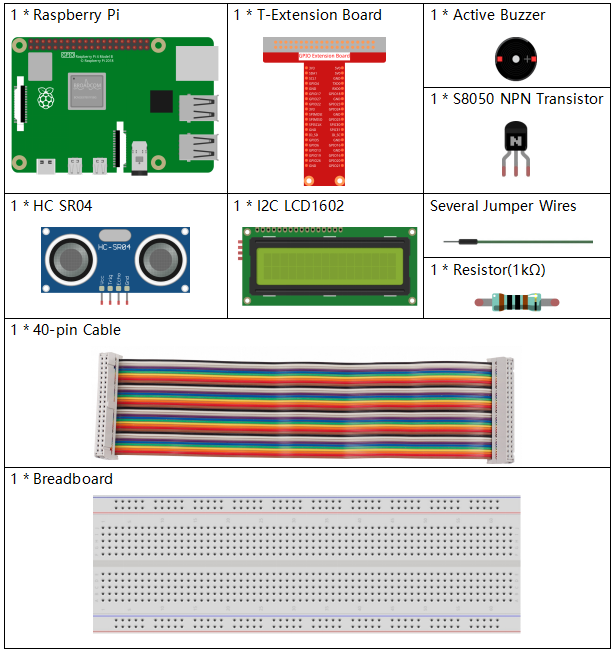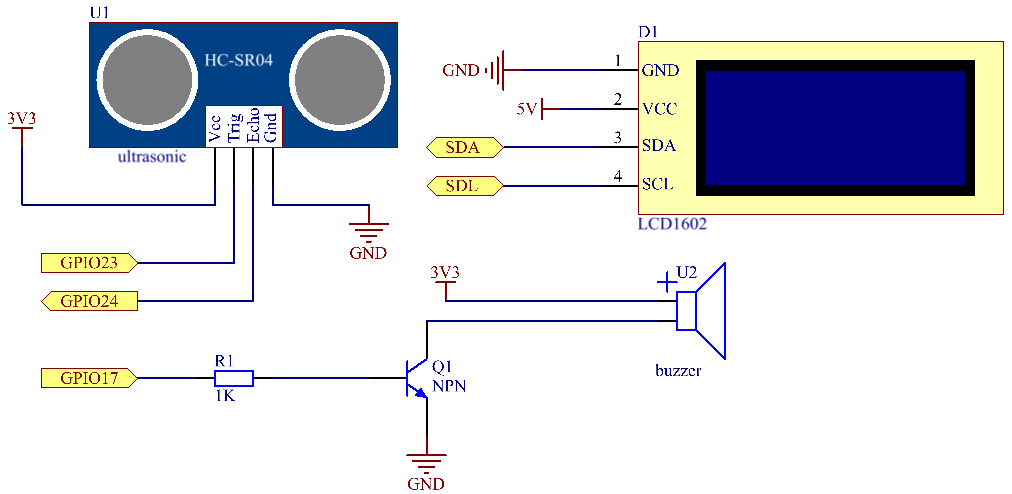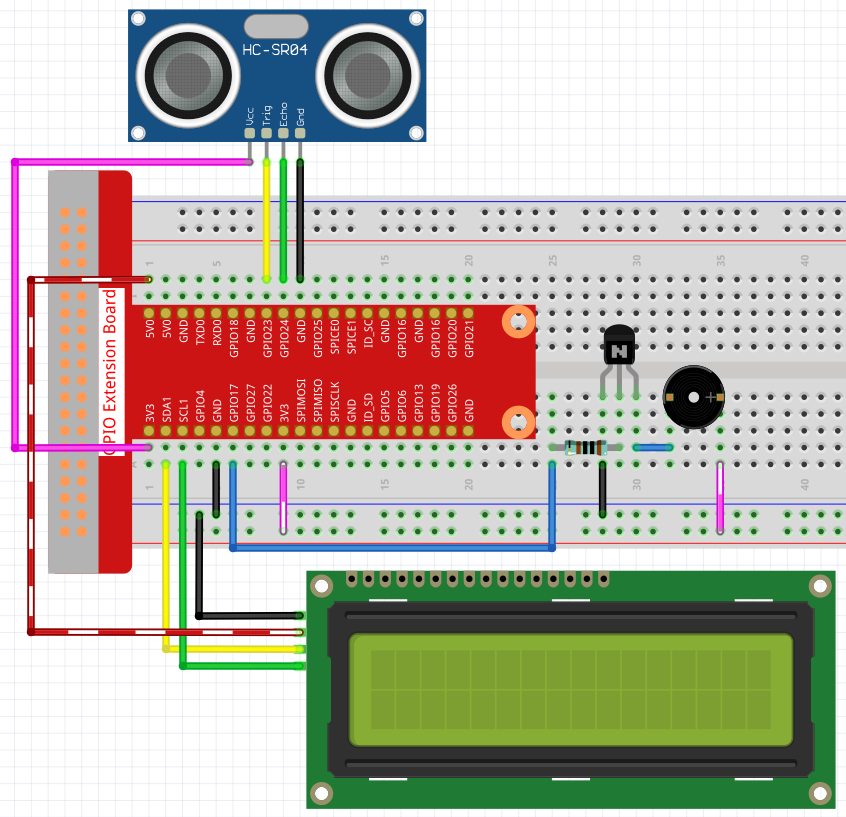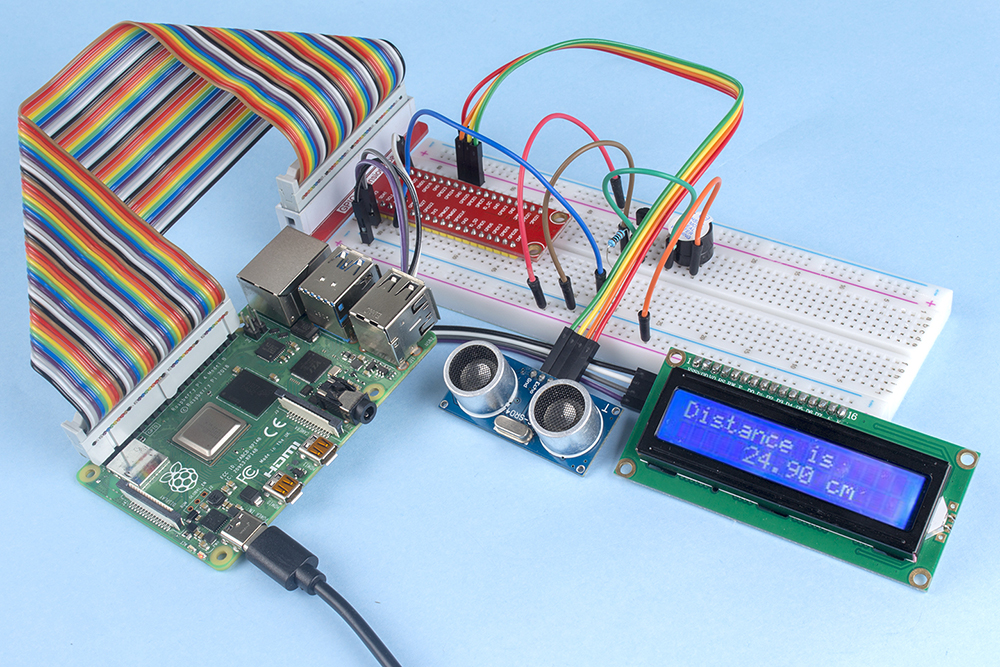# 4.1.9 Reversing Alarm¶

## Introduction¶

In this project, we will use LCD, buzzer and ultrasonic sensors to make a reverse assist system. We can put it on the remote control vehicle to simulate the actual process of reversing the car into the garage.

## Required Components¶

In this project, we need the following components.Name

ITEMS IN THIS KIT

Raphael Kit

337

Raphael Kit

COMPONENT INTRODUCTION

GPIO Extension Board

Jumper Wires

Resistor

Buzzer

-

Transistor

Ultrasonic Module

I2C LCD1602

## Schematic Diagram¶

Ultrasonic sensor detects the distance between itself and the obstacle that will be displayed on the LCD in the form of code. At the same time, the ultrasonic sensor let the buzzer issue prompt sound of different frequency according to different distance value.

 T-Board Name physical wiringPi BCM GPIO23 Pin 16 4 23 GPIO24 Pin 18 5 24 GPIO17 Pin 11 0 17 SDA1 Pin 3 SCL1 Pin 5## Experimental Procedures¶

Step 1: Build the circuit.Step 2: Change directory.

```cd ~/raphael-kit/python/
```

Step 3: Run.

```sudo python3 4.1.9_ReversingAlarm.py
```

As the code runs, ultrasonic sensor module detects the distance to the obstacle and then displays the information about the distance on LCD1602; besides, buzzer emits warning tone whose frequency changes with the distance.

Note

• If you get the error `FileNotFoundError: [Errno 2] No such file or directory: '/dev/i2c-1'`, you need to refer to I2C Configuration to enable the I2C.

• If you get `ModuleNotFoundError: No module named 'smbus2'` error, please run `sudo pip3 install smbus2`.

• If the error `OSError: [Errno 121] Remote I/O error` appears, it means the module is miswired or the module is broken.

• If the code and wiring are fine, but the LCD still does not display content, you can turn the potentiometer on the back to increase the contrast.

Code

Note

You can Modify/Reset/Copy/Run/Stop the code below. But before that, you need to go to source code path like `raphael-kit/python`. After modifying the code, you can run it directly to see the effect.

```import LCD1602
import time
import RPi.GPIO as GPIO

TRIG = 16
ECHO = 18
BUZZER = 11

def lcdsetup():
LCD1602.init(0x27, 1)   # init(slave address, background light)
LCD1602.clear()
LCD1602.write(0, 0, 'Ultrasonic Starting')
LCD1602.write(1, 1, 'By SunFounder')
time.sleep(2)

def setup():
GPIO.setmode(GPIO.BOARD)
GPIO.setup(TRIG, GPIO.OUT)
GPIO.setup(ECHO, GPIO.IN)
GPIO.setup(BUZZER, GPIO.OUT, initial=GPIO.LOW)
lcdsetup()

def distance():
GPIO.output(TRIG, 0)
time.sleep(0.000002)

GPIO.output(TRIG, 1)
time.sleep(0.00001)
GPIO.output(TRIG, 0)

while GPIO.input(ECHO) == 0:
a = 0
time1 = time.time()
while GPIO.input(ECHO) == 1:
a = 1
time2 = time.time()

during = time2 - time1
return during * 340 / 2 * 100

def destroy():
GPIO.output(BUZZER, GPIO.LOW)
GPIO.cleanup()
LCD1602.clear()

def loop():
while True:
dis = distance()
print (dis, 'cm')
print ('')
GPIO.output(BUZZER, GPIO.LOW)
if (dis > 400):
LCD1602.clear()
LCD1602.write(0, 0, 'Error')
LCD1602.write(3, 1, 'Out of range')
time.sleep(0.5)
else:
LCD1602.clear()
LCD1602.write(0, 0, 'Distance is')
LCD1602.write(5, 1, str(round(dis,2)) +' cm')
if(dis>=50):
time.sleep(0.5)
elif(dis<50 and dis>20):
for i in range(0,2,1):
GPIO.output(BUZZER, GPIO.HIGH)
time.sleep(0.05)
GPIO.output(BUZZER, GPIO.LOW)
time.sleep(0.2)
elif(dis<=20):
for i in range(0,5,1):
GPIO.output(BUZZER, GPIO.HIGH)
time.sleep(0.05)
GPIO.output(BUZZER, GPIO.LOW)
time.sleep(0.05)

if __name__ == "__main__":
setup()
try:
loop()
except KeyboardInterrupt:
destroy()
```

Code Explanation

```def lcdsetup():
LCD1602.init(0x27, 1)   # init(slave address, background light)

def setup():
GPIO.setmode(GPIO.BOARD)
GPIO.setup(TRIG, GPIO.OUT)
GPIO.setup(ECHO, GPIO.IN)
GPIO.setup(BUZZER, GPIO.OUT, initial=GPIO.LOW)
lcdsetup()
```

In this program, we apply the previously used components synthetically. Here we use buzzers, LCD and ultrasonic. We can initialize them in the same way as we did before.

```dis = distance()
print (dis, 'cm')
print ('')
GPIO.output(BUZZER, GPIO.LOW)
if (dis > 400):
LCD1602.clear()
LCD1602.write(0, 0, 'Error')
LCD1602.write(3, 1, 'Out of range')
time.sleep(0.5)
else:
LCD1602.clear()
LCD1602.write(0, 0, 'Distance is')
LCD1602.write(5, 1, str(round(dis,2)) +' cm')
```

Here we get the values of the ultrasonic sensor and get the distance through calculation. If the value of distance is greater than the range of value to be detected, an error message is printed on the LCD. And if the distance is within the working range, the corresponding results will be output.

```LCD1602.write(5, 1, str(round(dis,2)) +' cm')
```

Since the LCD output only supports character types, we need to use str () to convert numeric values to characters. We are going to round it to two decimal places.

```if(dis>=50):
time.sleep(0.5)
elif(dis<50 and dis>20):
for i in range(0,2,1):
GPIO.output(BUZZER, GPIO.HIGH)
time.sleep(0.05)
GPIO.output(BUZZER, GPIO.LOW)
time.sleep(0.2)
elif(dis<=20):
for i in range(0,5,1):
GPIO.output(BUZZER, GPIO.HIGH)
time.sleep(0.05)
GPIO.output(BUZZER, GPIO.LOW)
time.sleep(0.05)
```

This judgment condition is used to control the sound of the buzzer. According to the difference in distance, it can be divided into three cases, in which there will be different sound frequencies. Since the total value of delay is 500, all of them can provide a 500ms interval for the ultrasonic sensor to work.

## Phenomenon Picture¶